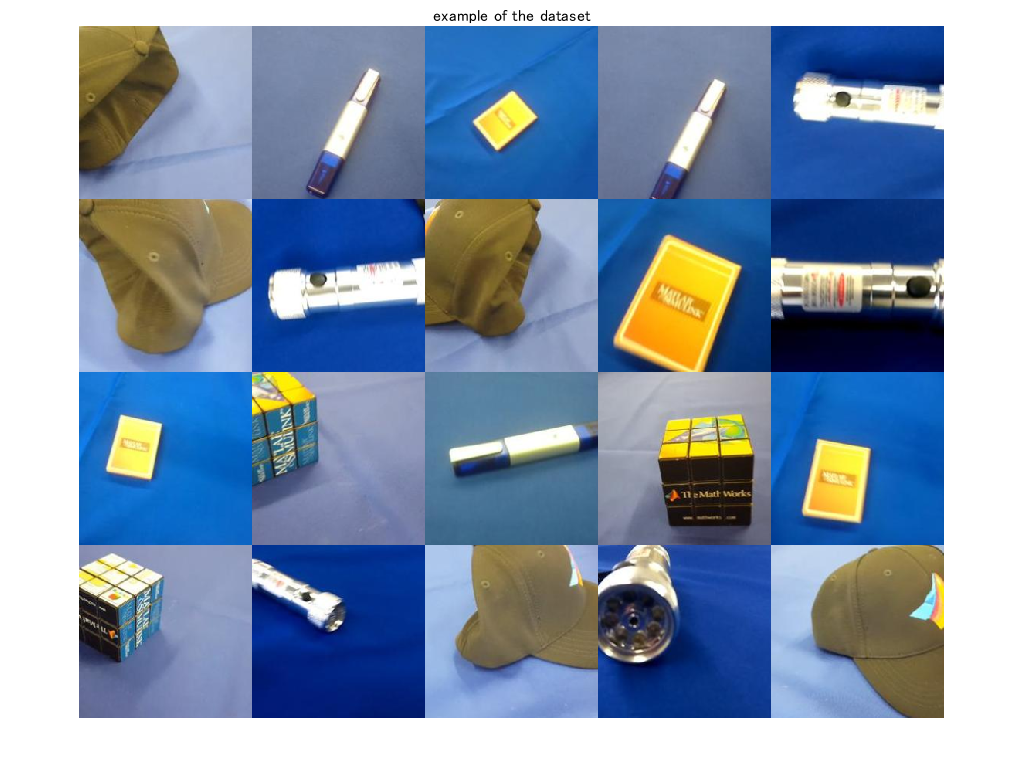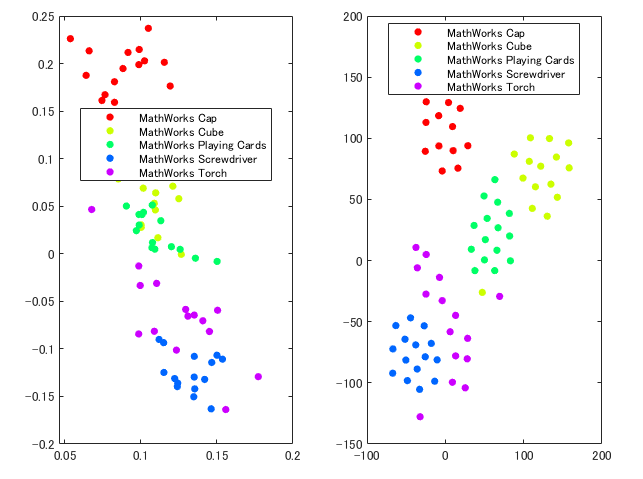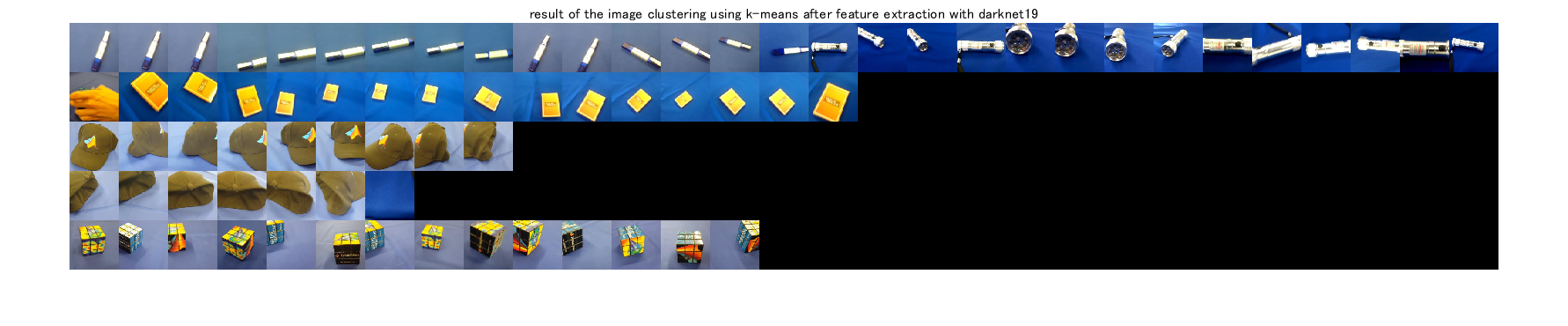## Image clustering and dimension reduction using CNN

Version 1.2 (2.33 MB) by
This demo shows how to perform image clustering and dimension reduction using a pre-trained network. 学習済みネットワークを利用し、画像のクラスタリングや次元圧縮を行います。

Updated 25 Jul 2021

From GitHub# Dimension reduction and clustering for images

[English]

This demo shows how to perform image clustering and dimension reduction using a pre-trained network. The network has learned rich feature representations for a wide range of images. The feature extraction with the pre-trained network can be utilized for image clustering.

This demo did

2. feature extraction with the network

3. dimension reduction with the extracted features

4. image clustering with k-means algorithm

The illustrations in the thumbnail were obtained from https://www.irasutoya.com/

[Japanese]

このデモでは、学習済みの深層学習ネットワークやクラスタリングアルゴリズムを用いて、画像の教師なし分類を行います。MathWorksの`MerchData`を用いて、`resnet-18`による特徴抽出の後に、k-meansアルゴリズムにより分類を行います。

# Preparation

• We use a dataset called MearchData provided by Mathworks
• Load a pre-trained network called darknet19
• Load images from the dataset
``````clear;clc;close all
% unzip the zip file of MearchData
unzip('MerchData.zip');
``````

import a pre-trained network called `resnet18`

``````net=resnet18;
% load the images into the image data store called imds
imds = imageDatastore('MerchData','IncludeSubfolders',true,'LabelSource','foldernames');
% use augmented image datastore for image augmentation
augImds=augmentedImageDatastore(net.Layers(1, 1).InputSize(1:2),imds);
``````

Display some images from the dataset

``````% randomly extract image index to display some images
idx=randperm(numel(imds.Files),20);
% to show the tiled images
figure;montage(imgEx.input);title('example of the dataset')
``````Feature extraction using a pre-trained network

``````% Gather label information from the image datastore
Labels=imds.Labels;
% count the number of images
numClass=numel(countcats(Labels));
% feature extraction with the pre-trained network
feature=squeeze(activations(net,augImds,'fc1000'));
``````

# Dimension reduction with Principal component analysis and t-sne

``````figure;
% conduct a principal component analysis for the dimension reduction
A=pca(feature,"Centered",true);
subplot(1,2,1)
gscatter(A(:,1),A(:,2),Labels)
subplot(1,2,2)
% perform t-sne for the dimension reduction
T=tsne(feature');
gscatter(T(:,1),T(:,2),Labels)
``````# Image clustering using k-means after feature extraction with resnet-18

``````% perform k-means algorithm
% please note that as the result is dependent on the initial point in the algorithm, the
% result would not be same
C=kmeans(feature',numClass,"Start","plus");
% confirm the number of images in the largest group
[~,Frequency] = mode(C);
sz=net.Layers(1, 1).InputSize(1:2);
% prepare a matrix to show the clustering result
I=zeros(sz(1)*numClass,sz(2)*Frequency,3,'uint8');
% loop over the class to display images assigned to the group
for i=1:numClass
% read the images assigned to the group
% use the function "find" to find out the index of the i-th group image
% tile the images extracted above
I((i-1)*sz(1)+1:i*sz(1),1:sz(2)*numel(find(C==i)),:)=cat(2,ithGroup.input{:});
end
figure;imshow(I);title('result of the image clustering using k-means after feature extraction with darknet19')
``````### Cite As

Kenta (2023). Image clustering and dimension reduction using CNN (https://github.com/KentaItakura/Dimension-reduction-and-clustering-for-images-using-MATLAB/releases/tag/1.2), GitHub. Retrieved .

##### MATLAB Release Compatibility
Created with R2020a
Compatible with any release
##### Platform Compatibility
Windows macOS Linux

### Community Treasure Hunt

Find the treasures in MATLAB Central and discover how the community can help you!

Start Hunting!
Version Published Release Notes
1.2

See release notes for this release on GitHub: https://github.com/KentaItakura/Dimension-reduction-and-clustering-for-images-using-MATLAB/releases/tag/1.2

1.0.2